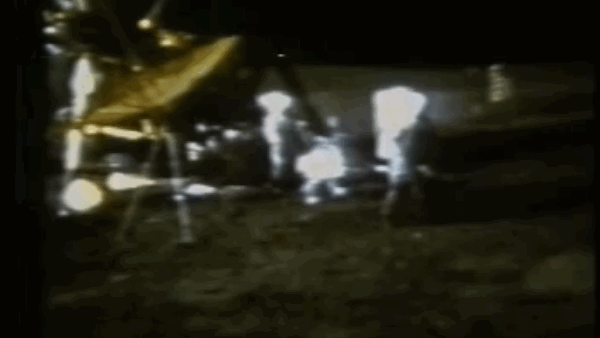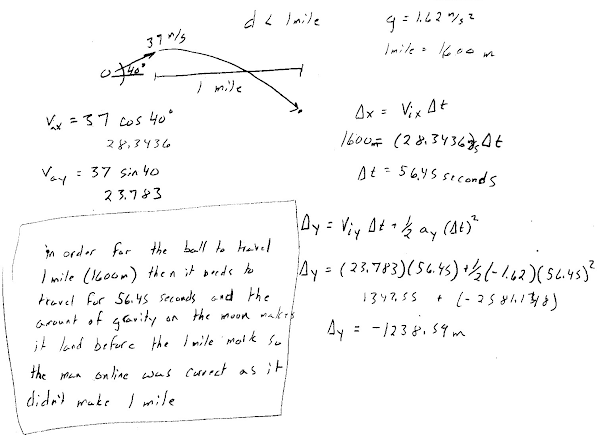## 20141019

### Physics midterm problem: Apollo 14 golf swing

Physics 205A Midterm 1, fall semester 2014
Cuesta College, San Luis Obispo, CA"Golf on the Moon (NASA)"
youtu.be/KZLl3XwlAIE

In February 1971, Apollo 14 astronaut Alan Shepard used a make-shift golf club to hit a golf ball on the moon, and described it as traveling "miles and miles and miles."[*] An online message board[**] discussed reasonable parameters for a golf ball hit by an average golfer:
I am informed by a mathematician, also a keen golfer, that assuming a speed off the club of 37 m/s, which is average, and an elevation of 40°, which is usual for a 6-iron, then a distance [less than one mile] could be expected [on the moon].
Based on these parameters, determine whether Shepard's "miles and miles and miles" claim or the online "less than one mile" claim is more plausible. The magnitude of the gravitational constant on the moon is gmoon = 1.62 m/s2, and use the approximation 1 mi = 1,600 m. Neglect air resistance. Show your work and explain your reasoning using properties of projectile motion.

[*] Eric M. Jones, "EVA-2 Closeout and the Golf Shots," history.nasa.gov/alsj/a14/a14.clsout2.html.
[**] moorouge, "Topic: How far did Alan Shepard's golf ball go?" collectspace.com/ubb/Forum29/HTML/001181.html.

• p:
Correct. Finds x- and y-components of initial velocity: v0x = +28 m/s, v0y = +24 m/s; then applies projectile motion equations in a methodical manner and determines which claim is more plausible using one of these (or other) approaches:
• finds t for the golf ball to reach the ground, then uses this time to determine the final horizontal position x of the ball, which is less than 1,600 m;
• finds time t for the golf ball to theoretically travel to a final horizontal position x = +1,600 m, then uses this time to determine the final vertical position y of the golf ball, and concludes that since y is negative, then the golf ball must have traveled less than 1,600 m.
• r:
Nearly correct, but includes minor math errors. May have the wrong sign on the gravitational acceleration constant, or simple arithmetic errors, but makes a sound argument based on the numerical values resulting from these errors.
• t:
Nearly correct, but approach has conceptual errors, and/or major/compounded math errors. At least enough steps are shown that would theoretically result in a complete answer, multiple errors notwithstanding.
• v:
Implementation of right ideas, but in an inconsistent, incomplete, or unorganized manner.
• x:
Implementation of ideas, but credit given for effort rather than merit.
• y:
Irrelevant discussion/effectively blank.
• z:
Blank.
Sections 70854, 70855, 73320
Exam code: midterm01m0oU
p: 23 students
r: 15 students
t: 8 students
v: 8 students
x: 14 students
y: 5 students
z: 1 student

A sample "p" response (from student 3560), calculating the time for the golf ball to hit the ground, and finding that the horizontal distance traveled during that time would be less than a mile:Another sample "p" response (from student 0123), calculating the time for the golf ball to travel one mile horizontally, then determining that the golf ball would need to be vertically well below the ground in order to travel that horizontal distance:Another sample "p" response (from student 1263), calculating the time for the golf ball to reach its highest height, then multiplying that by two to get the total flight time, and finding that the horizontal distance traveled during that total flight time would be less than a mile:A sample "y" response (from student 9693), speculating that the golf ball would travel farther than a mile on Earth because of the weaker gravitational constant on the moon.